GeeksforGeeks App
Open AppBrowser
Continue

# Class 10 NCERT Solutions- Chapter 3 Pair of Linear Equations in Two Variables – Exercise 3.6

###Solution:

Lets, take 1/x = a and 1/y = b

Here, the two given equation will be as follows:+= 2

Multiply it by 6, we get

3a + 2b = 12            -(1)

and,+=Multiply it by 6, we get

2a + 3b = 13            -(2)

Now, by using Elimination method,

Multiply eq(1) by 2 and multiply eq(2) by 3, and then subtract them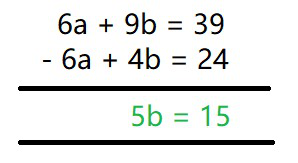5b = 15

b = 3

Now putting b = 3 in eq(1), we get

3a + 2(3) = 12

a = 6/3

a = 2

So, Now As

a = 1/x = 2

x = 1/2

b = 1/y = 3

y = 1/3

###Solution:

Lets, take 2/√x = a and 3/√y = b

Here, the two given equation will be as follows:

a + b = 2            -(1)

and,

2a – 3b =-1            -(2)

Now, by using Elimination method,

Multiply eq(1) by 3, and then add them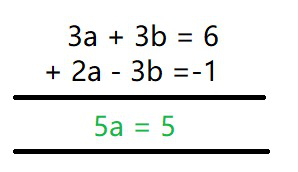5a = 5

a = 1

Now putting a = 1 in eq(1), we get

1 + b = 2

b = 1

So, Now As

a = 2/√x = 1

√x = 2

x = 4

b = 3/√y = 1

√x = 3

y = 9

###– 4y = 23

Solution:

Lets, take 1/x = a

Here, the two given equation will be as follows:

4a + 3y = 14            -(1)

and,

3a – 4y = 23             -(2)

Now, by using Elimination method,

Multiply eq(1) by 3 and multiply eq(2) by 4, and then subtract them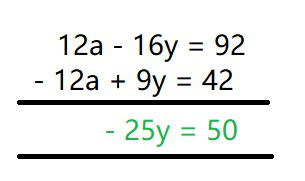-25y = 50

y = -2

Now putting y = -2 in eq(1), we get

4a + 3(-2) = 14

4a = 20

a = 5

So, Now As

a = 1/x  = 5

x = 1/5

y = -2

###Solution:

Lets, take= a and,= b

Here, the two given equation will be as follows:

5a + b = 2            -(1)

and,

6a – 3b = 1             -(2)

Now, by using Elimination method,

Multiply eq(1) by 3, and then add them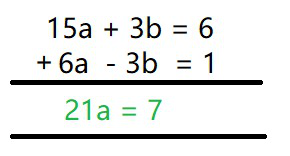21a = 7

a = 1/3

Now putting a = 1/3  in eq(1), we get

5(1/3) + b = 2

b = 2 – 5/3

b = 1/3

So, Now As

a =x – 1 = 3

x = 4

b =y – 2 = 3

y = 5

###Solution:= 5= 15

Lets, take 1/x = a and 1/y = b

Here, the two given equation will be as follows:

7b – 2a = 5             -(1)

and,

8b + 7a = 15             -(2)

Now, by using Elimination method,

Multiply eq(1) by 7, multiply eq(2) by 2 and then add them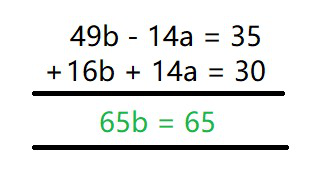65b = 65

b = 1

Now putting b = 1 in eq(1), we get

7(1) – 2a = 5

2a = 7 – 5

a = 1

So, Now As

a = 1/x = 1

x = 1

b = 1/y = 1

y = 1

### 2x + 4y = 5xy

Solution:

Divide both the equations by xy, we get= 6= 5

Lets, take 1/x = a and, 1/y = b

Here, the two given equation will be as follows:

6b + 3a = 6

Divide the above equation by 2,

2b + a = 2             -(1)

and,

2b + 4a = 5             -(2)

Now, by using Elimination method,

Subtract eq(1) from eq(2), we get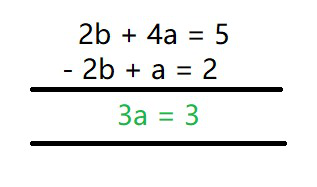3a = 3

a = 1

Now putting a = 1 in eq(1), we get

2b + 1 = 2

b = 1/2

So, Now As

a = 1/x = 1

x = 1

b = 1/y = 1/2

y = 2

###Solution:

Lets, take= a and= b

Here, the two given equation will be as follows:

10a + 2b = 4

Divide the above equation by 2,

5a + b = 2             -(1)

and,

15a – 5b = -2             -(2)

Now, by using Elimination method,

Multiply eq(1) by 3 and subtract them,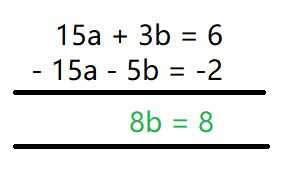8b = 8

b = 1

Now putting b = 1 in eq(1), we get

5a + 1 = 2

a = 1/5

So, Now As

a ==x + y = 5             -(3)

b == 1

x – y = 1             -(4)

By adding eq(3) and (4), we get

2x = 6

x = 3 and y = 2

###Solution:

Lets, take= a

and,= b

Here, the two given equation will be as follows:

a + b = 3/4           -(1)

and,Multiply it by 2, we get

a – b = -1/4           -(2)

Now, by using Elimination method,

Add eq(1) and eq(1), we get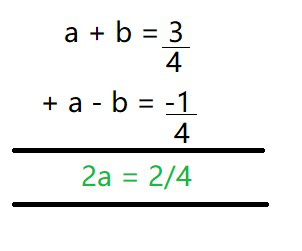2a = 1/2

a = 1/4

Now putting a = 1/4 in eq(1), we get+ b =b = 1/2

So, Now As

a =3x + y = 4           -(3)

b =3x – y = 2            -(4)

By adding eq(3) and eq(4), we get

6x = 6

x = 1 and y = 1

### (i) Ritu can row downstream 20 km in 2 hours, and upstream 4 km in 2 hours. Find her speed of rowing in still water and the speed of the current.

Solution:

Let us consider,

Speed of Ritu in still water = x km/hr

Speed of Stream = y km/hr

Now, speed of Ritu during,

Downstream = (x + y) km/h

Upstream = (x – y) km/h

As Speed =According to the given question,

x + y = 20/2

x + y = 10           -(1)

and,

x – y = 4/2

x – y = 2            -(2)

Add eq(1) and eq(2), we get

2x = 12

x = 6 and y = 4

Hence, speed of Ritu rowing in still water = 6 km/hr

Speed of Stream = 4 km/hr

### (ii) 2 women and 5 men can together finish an embroidery work in 4 days, while 3 women and 6 men can finish it in 3 days. Find the time taken by 1 woman alone to finish the work, and also that taken by 1 man alone.

Solution:

Let’s take,

The total number of days taken by women to finish the work = x

The total number of days taken by men to finish the work = y

Work done by women in one day will be = 1/x

Work done by women in one day will be = 1/y

So, according to the question

4() = 1

And, 3() = 1

Lets, take 1/x = a and,  1/y = b

Here, the two given equation will be as follows:

4(2a + 5b) = 1

8a + 20b = 1            -(1)

and,

3(3a + 6b) = 1

9a + 18b = 1            -(2)

Now, by using Cross multiplication method,a =b = 1/36

So, Now As

a =x = 18

b =y = 36

Hence, number of days taken by women to finish the work = 18 days

Number of days taken by men to finish the work = 36 days.

### (iii) Roohi travels 300 km to her home partly by train and partly by bus. She takes 4 hours if she travels 60 km by train and the remaining by bus. If she travels 100 km by train and the remaining by bus, she takes 10 minutes longer. Find the speed of the train and the bus separately.

Solution:

Lets, take

Speed of the train = x km/h

Speed of the bus = y km/h

According to the given question,= 4Lets, take 1/x = a and 1/y = b

Here, the two given equation will be as follows:

60a + 240b = 4

Divide it by 4, we get

15a + 60b = 1           -(1)

and,

100a + 200b = 25/6

Divide it by 25/6, we get

24a + 48b = 1           -(2)

Now, by using Cross multiplication method,a ==b ==So, Now As

a ==x = 60

b ==y = 80

Hence, speed of the train = 60 km/h

Speed of the bus = 80 km/h

My Personal Notes arrow_drop_up
Related Tutorials